# Kinematic Equations NIS grade 11 physics reviewPage 3

#### WATCH ALL SLIDES

Slide 21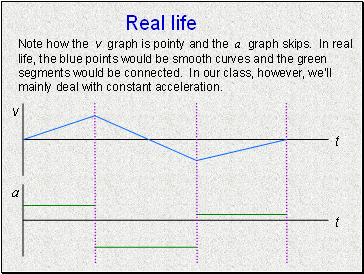## Real life

Note how the v graph is pointy and the a graph skips. In real life, the blue points would be smooth curves and the green segments would be connected. In our class, however, we’ll mainly deal with constant acceleration.

Slide 22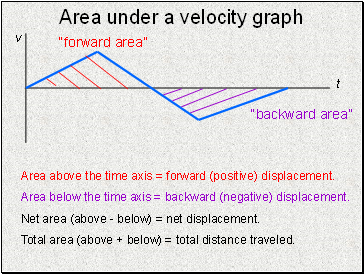Area under a velocity graph

“forward area”

“backward area”

Area above the time axis = forward (positive) displacement.

Area below the time axis = backward (negative) displacement.

Net area (above - below) = net displacement.

Total area (above + below) = total distance traveled.

Slide 23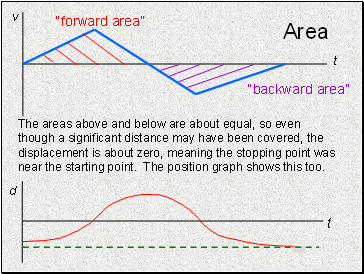## Area

The areas above and below are about equal, so even though a significant distance may have been covered, the displacement is about zero, meaning the stopping point was near the starting point. The position graph shows this too.

Slide 24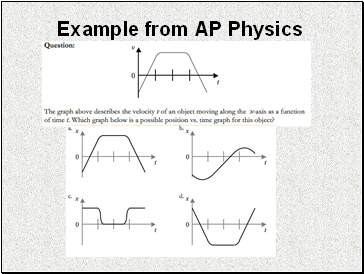## Example from AP Physics

Slide 25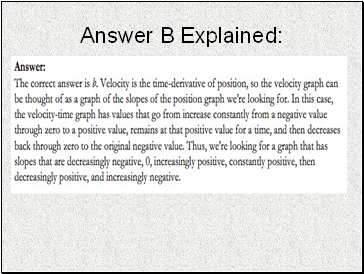Slide 26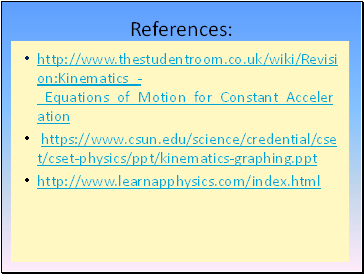References:

http://www.thestudentroom.co.uk/wiki/Revision:Kinematics_-_Equations_of_Motion_for_Constant_Acceleration

https://www.csun.edu/science/credential/cset/cset-physics/ppt/kinematics-graphing.ppt

http://www.learnapphysics.com/index.html

Go to page:
1  2  3